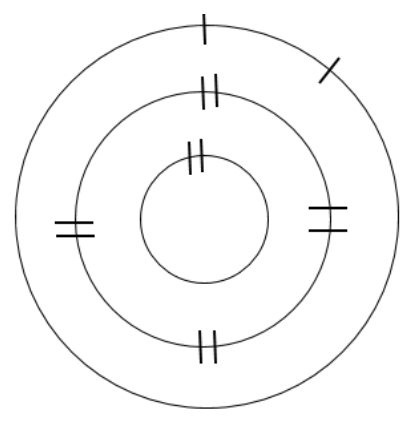# 38.4: Procedures

$$\newcommand{\vecs}{\overset { \rightharpoonup} {\mathbf{#1}} }$$ $$\newcommand{\vecd}{\overset{-\!-\!\rightharpoonup}{\vphantom{a}\smash {#1}}}$$$$\newcommand{\id}{\mathrm{id}}$$ $$\newcommand{\Span}{\mathrm{span}}$$ $$\newcommand{\kernel}{\mathrm{null}\,}$$ $$\newcommand{\range}{\mathrm{range}\,}$$ $$\newcommand{\RealPart}{\mathrm{Re}}$$ $$\newcommand{\ImaginaryPart}{\mathrm{Im}}$$ $$\newcommand{\Argument}{\mathrm{Arg}}$$ $$\newcommand{\norm}{\| #1 \|}$$ $$\newcommand{\inner}{\langle #1, #2 \rangle}$$ $$\newcommand{\Span}{\mathrm{span}}$$ $$\newcommand{\id}{\mathrm{id}}$$ $$\newcommand{\Span}{\mathrm{span}}$$ $$\newcommand{\kernel}{\mathrm{null}\,}$$ $$\newcommand{\range}{\mathrm{range}\,}$$ $$\newcommand{\RealPart}{\mathrm{Re}}$$ $$\newcommand{\ImaginaryPart}{\mathrm{Im}}$$ $$\newcommand{\Argument}{\mathrm{Arg}}$$ $$\newcommand{\norm}{\| #1 \|}$$ $$\newcommand{\inner}{\langle #1, #2 \rangle}$$ $$\newcommand{\Span}{\mathrm{span}}$$$$\newcommand{\AA}{\unicode[.8,0]{x212B}}$$

You will search for patterns of electron configurations.

1. Choose one Group A set of elements (one of the Group A columns) for which you will be responsible.  Each person on the team should have one Group A column, initially.

2. Sketch the number of shells, or orbits, your first element would have in the ground state.  Use hash marks to show the correct number of pairs for each inner orbit.  Count the number of electrons added to the inner orbits, and calculate the number of electrons remaining.

Example:

• Magnesium (Mg) has atomic number 12, so it will normally have 12 electrons

• (12 electrons total)  − (10 electrons added to shells)  =  2 electrons remainingFigure $$\PageIndex{1}$$
1. Determine the number of electrons needed to fill the outermost shell half full.  Add the remaining electrons as singles, until the shell is half full, and then pair electrons (if needed) until all electrons for that element have been added to the model.Figure $$\PageIndex{2}$$
1. Repeat the process for each element in your first Group A column.  Determine and record the number of unpaired electrons in the outermost shell, for your first set of Group A elements; each of these elements should have the same trend (except Group IIIA – the trend changes).  Also record whether the outermost shell is mostly full or empty.

2. Choose a 2nd Group A set of elements (another Group A column), and complete the entire process for that set of elements.

3. Draw a table in which to record the trend for each vertical group of elements.  Read the instructions for completing the table.

Table $$\PageIndex{1}$$: Periodic Table Trends

Group

Unpaired Electrons Trend

Mostly (full/empty)

IA

IIA

IIIA

IVA

VA

VIA

VIIA

VIIIA

1. Collaborate with your team to record the trends for each Group A column (IA, IIA, IIIA, IVA, VA, VIA, VIIA, VIIIA).  Record the number of unpaired electrons in the outermost shell, and record whether the outermost shell is mostly empty or mostly full.